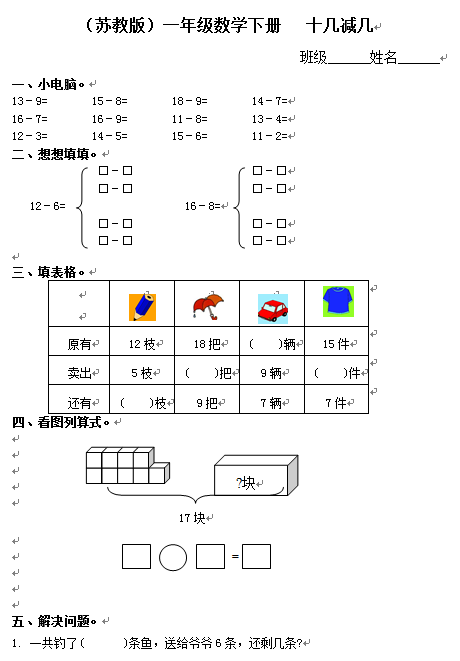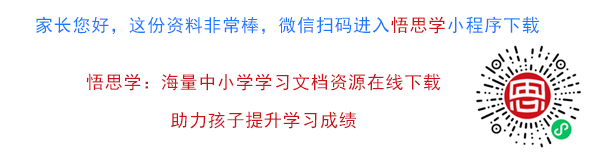（苏教版）一年级数学下册   十几减几

13－9=       15－8=       18－9=       14－7=

16－7=       16－9=       11－8=       13－4=

12－3=       14－5=       15－6=       11－2=

【DOC文档4页】（苏教版）一年级数学下册 十几减几及答案 2 A4电子版资料_可直接打印_会员免费下载##### 评论信息